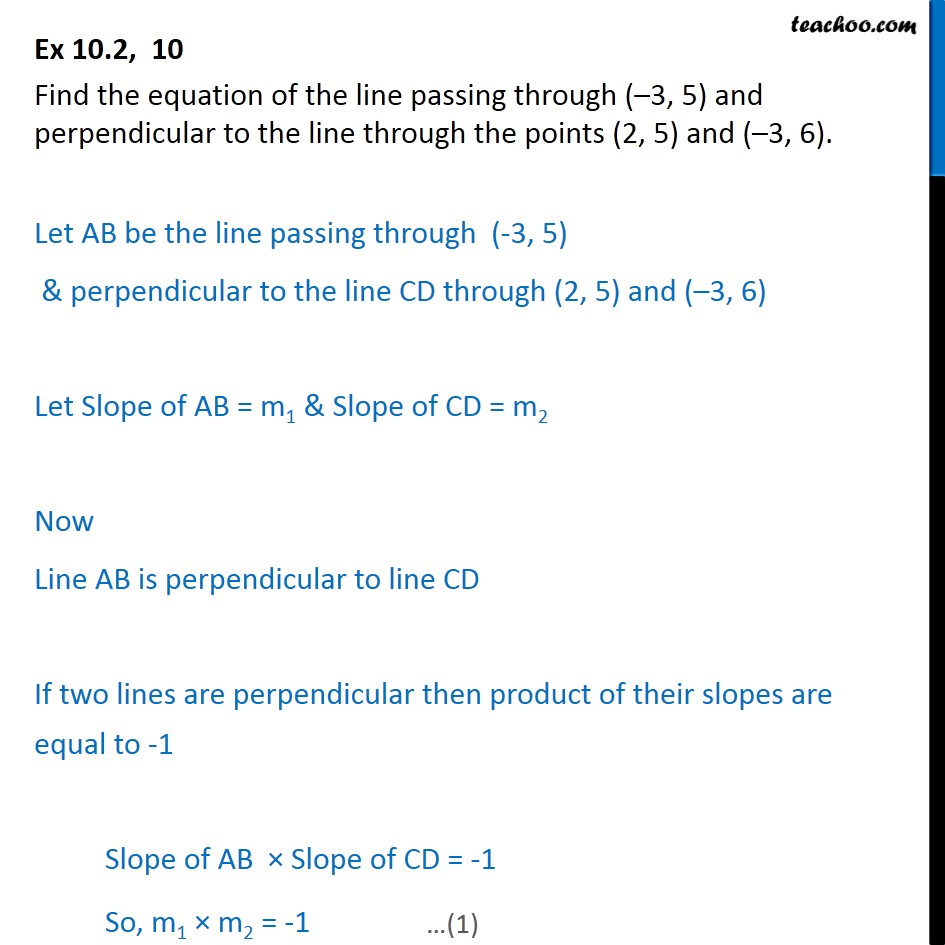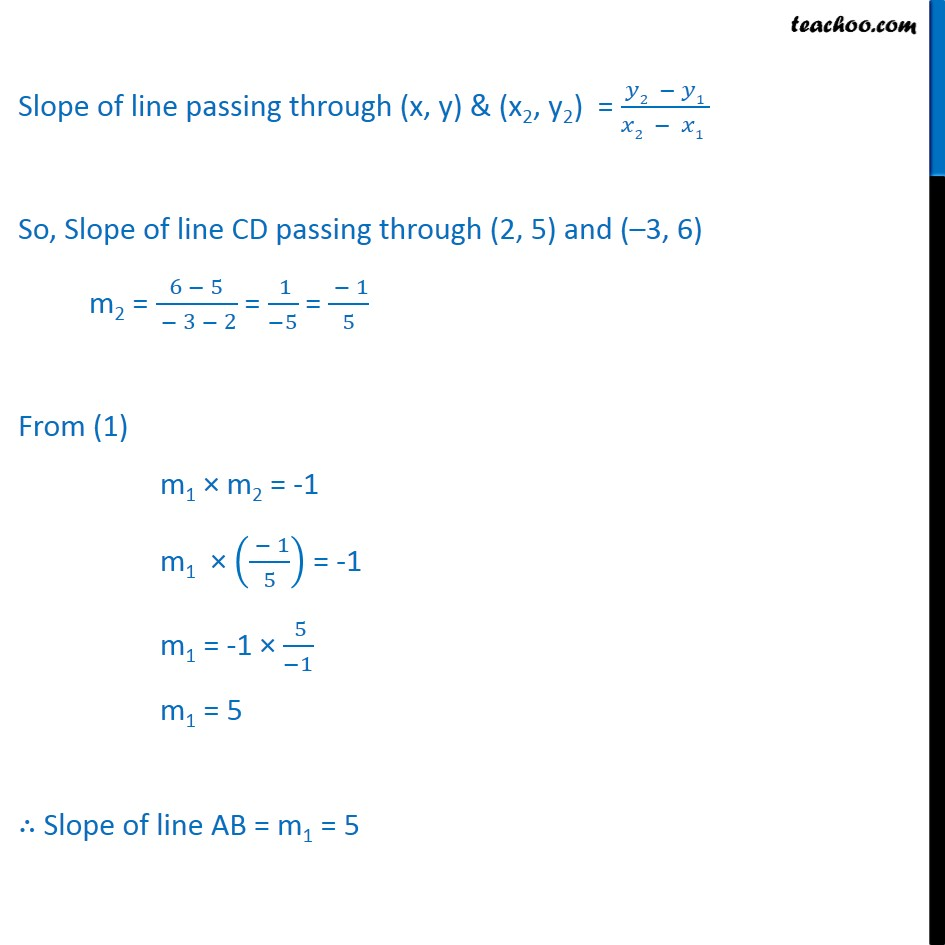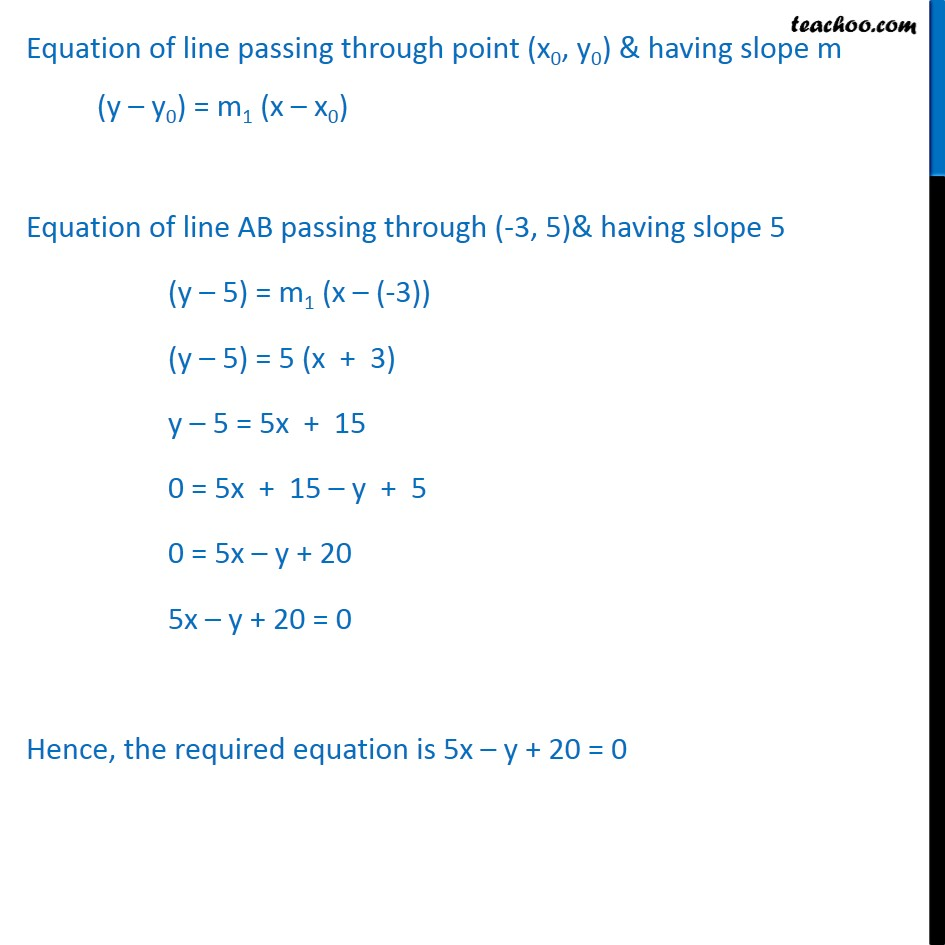Ex 9.2

Chapter 9 Class 11 Straight Lines
Serial order wiseLearn in your speed, with individual attention - Teachoo Maths 1-on-1 Class

### Transcript

Ex 9.2, 10 Find the equation of the line passing through ( 3, 5) and perpendicular to the line through the points (2, 5) and ( 3, 6). Let AB be the line passing through (-3, 5) & perpendicular to the line CD through (2, 5) and ( 3, 6) Let Slope of AB = m1 & Slope of CD = m2 Now Line AB is perpendicular to line CD If two lines are perpendicular then product of their slopes are equal to -1 Slope of AB Slope of CD = -1 So, m1 m2 = -1 Slope of line passing through (x, y) & (x2, y2) = ( 2 1)/( 2 1 ) So, Slope of line CD passing through (2, 5) and ( 3, 6) m2 = (6 5)/( 3 2) = ( 1)/( 5) = ( 1)/5 From (1) m1 m2 = -1 m1 (( 1)/5) = -1 m1 = -1 ( 5)/( 1) m1 = 5 Slope of line AB = m1 = 5 Equation of line passing through point (x0, y0) & having slope m (y y0) = m1 (x x0) Equation of line AB passing through (-3, 5)& having slope 5 (y 5) = m1 (x (-3)) (y 5) = 5 (x + 3) y 5 = 5x + 15 5x + 15 y + 5 = 0 5y y + 20 = 0 Hence, the required equation is 5y y + 20 = 0